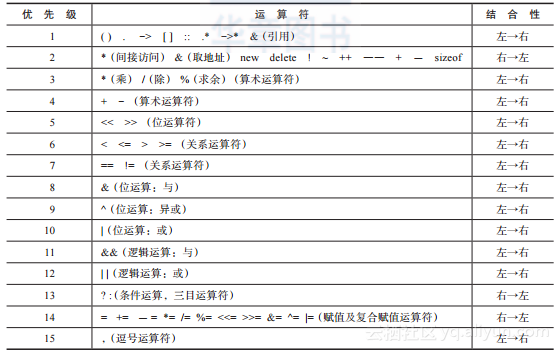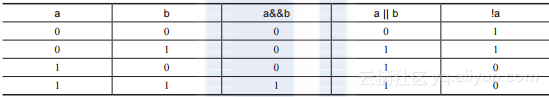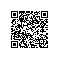# 《C++程序设计教程（第3版）》——第2章，第4节基本运算符和表达式

2.4　基本运算符和表达式
2.4.1　C++运算符及表达式简介2.4.2　算术运算符和算术表达式
C++提供了5个算术运算符，它们是+（加）、-（减）、*（乘）、/（除）、%（求余），都是二元运算符，其中+（正号）和-（负号）又可用作一元运算符。

2.4.3　运算优先级和结合性
C++表达式中可以出现多个运算符和运算量，计算表达式时必须按照一定的次序，运算符的优先级和结合性规定了运算次序。

2.4.4　关系运算符和关系表达式
“关系运算符”实际上就是“比较运算符”。关系运算符的意义及其运算优先次序如下：

2.4.5　逻辑运算符和逻辑表达式
C++语言中提供了3个逻辑运算符，如下所示。
！ 逻辑“非”（一元运算符）
&& 逻辑“与”（二元运算符）
|| 逻辑“或”（二元运算符）C++在给出关系表达式或逻辑表达式运算结果时，以数值1代表“真”，以数值0代表“假”。例如，若a＝1、b＝-2，则表达式a＞b的值为1。

2.4.6　位运算符和位运算表达式

1.按位与（&）
；再计算0运算符“&”将两个运算量的对应二进制位逐一按位进行逻辑与运算。每个二进制位（包括符号位）均参加运算。例如：
char a=3, b=-2, c; // 此时，可将a、b、c看成1字节长度的整型量
 c = a & b;

       a  0000 0011
&  b  1111 1110
c  0000 0010

2.按位或（|）

char a=18, b=3, c; // 此时，可将a、b、c看成1字节长度的整型量
 c = a | b ;

       a  0001 0010
|  b  0000 0011
c  0001 0011

3.按位异或（^）

char a=18, b=3, c; // 此时，可将a、b、c看成1字节长度的整型量
 c = a ^ b ;

   a  0001 0010

^ b 0000 0011

   c  0001 0001

int a=5, b=9;
a = a^b;
b = a^b;
a = a^b;
4.按位取反（~）

int a=18, b;

  b = ~a;

~ a 0000 0000 0000 0000 0000 0000 0001 0010

  b  1111 1111 1111 1111 1111 1111 1110 1101

5.左移（<<）

6.右移（>>）

char a = -4, b=4, x, y;
x = a >> 2;
y = b >> 2;
a: 1111 1100 → x: 1111 1111
b: 0000 0100 → y: 0000 0001
x的值是-1，y的值是1。右移一位相当于除以2操作。又例如：
unsigned char a = -4 , x ;
x = a >> 2;
a: 1111 1100 → x: 0011 1111
x的值是63。此时，右移一位符号位发生了变化，称为溢出，就不表示除2操作了。

int a=1, b;
b = a+2;

2.4.7　自增、自减运算符和表达式
C++中有两个可以改变变量自身值的运算符，它们是自增（++）和自减（--）运算符，作用是使变量自身的值加1或减1。这两个运算符均是一元运算符，而且只能对变量做运算。它们可以放在变量之前或之后，如++i、--i表示先将i的值加1或减1，然后再参加其他运算；而i++、i--表示先用i的值参加运算，然后再将变量i的值加1或减1。

2.4.8　赋值运算符和赋值表达式
1.赋值运算符

<变量> <赋值运算符> <表达式>

2.复合赋值运算符

+= －= *= /= %= <<= >>= &= ^= |=
“复合赋值运算符”与“赋值运算符”的优先级和结合性是一样的。如y=x+8，等价于y=(x+8)，也等价于y=y(x+8)。又如表达式a+=a-=aa，如果a的初值为2，则先计算a*a，值为4，再计算a-=4，结果a的值变成-2，同时表达式a-=4的值也是-2，再计算a+=(-2)，结果a的值是-4，整个表达式的值也是-4。

2.4.9　逗号运算符和逗号表达式

<表达式1> ,<表达式2>,…,<表达式n>

a=35, a4 //是逗号表达式，运算结束后a=15，整体表达式的值为60
x=(a=3, 6*3) //是赋值表达式，运算结束后a=3，x=18，整体表达式的值为18
x=a=3, 6*3 //是逗号表达式，运算结束后a=3，x=3，整体表达式的值为18

2.4.10　sizeof 运算符和表达式
sizeof运算符是一元运算符，它的作用是求一个变量或常量所占用的字节数。其格式为：
sizeof (<类型标识>/<变量名>)

2.4.11　逻辑运算优化的副作用
C++语言在计算逻辑表达式的值时，从左向右扫描表达式，表达式的值一旦确定后，就不再继续进行计算，这就是逻辑运算的优化。具体表现如下所示。

int x, y, z, w;
x = y = z = 1 ;
w = ++x || ++y && ++z; // A

int x = -1, y = 2, z = 0, w;
w = ++x && ++y || ++z; // B